## In an experiment, an object is released from rest from the top of a building. Its speed is measured as it reaches a point that is a distance

Question

In an experiment, an object is released from rest from the top of a building. Its speed is measured as it reaches a point that is a distance d from the point of release. If this distance was doubled, what would the new speed be, assuming air resistance is negligible?

in progress 0
3 weeks 2021-08-23T22:55:34+00:00 1 Answers 0 views 0

so the speed will increase by 1.44 times then the initial speed if the distance is increased to double

Explanation:

As we know that the air friction or resistance due to air is neglected then we can use the equation of kinematics here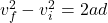since we released it from rest so we have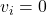so here we have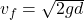now if the distance is double then we have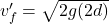now from above two equations we can say that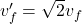so the speed will increase by 1.44 times then the initial speed if the distance is increased to double